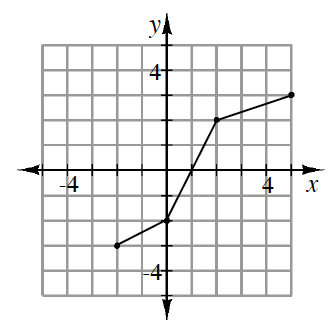### Home > INT3 > Chapter 5 > Lesson 5.1.2 > Problem5-34

5-34.

The function $f(x)$ is represented in the graph at right. Draw a graph of its inverse function. Be sure to state the domain and range for both $f(x)$ and $f^{–1}(x)$. What is the relationship between the domain and range of the original function and the domain and range of the inverse?

Switch the coordinates of the points on the graph to draw the inverse.Use the eTool below to graph the inverse function.
Click the link at right for the full version of the eTool: 5-34 HW eTool